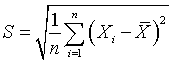# Can the standard deviation be more than half of the range?

## 2007-06-22

### Dear Professor Mean,

I was trying to work with some simple data sets to see how large I could make the standard deviation relative to the range. I know the standard deviation can never be larger than the range, but I can’t seem to get it to be larger than half the range.*

There are some accountants who used to work with Enron who can do some very creative things with numbers. Have you tried talking to them?

It helps to look at the formula. The most commonly used formula isbut some people useinstead. If you use the second formula, then it is pretty obvious that the standard deviation cannot exceed the range. The mean of the data has to be inside the range of the data, so no single term (before being squared) in the sum can exceed the range. This makes it easy to show that the standard deviation has to be smaller than the range.

If the data is symmetric, you can say even more. The mean is exactly in the middle of the range. Each term in the sum is less than or equal to half the range, therefore the standard deviation is less than or equal to half the range.

You can probably show that the standard deviation is less than or equal to half the range for asymmetric data as well, but the mathematics is beyond my grasp on a Friday afternoon.

You can achieve the maximum standard deviation for a very simple case. Select two data points: 0 and 1 and compute the standard deviation using the second formula. It will be 0.5, which is exactly half the range.

Now when you use the first formula, you will get a slightly larger value. In fact the ratio of the first formula to the second formula is simplywhich is maximized for n=2. We won’t talk about the case where n=1. So the best case (two data points 0 and 1) yields a standard deviation of 0.7071 which is more than 50% of the range. I don’t think you can do any better than this.

Oh yes, there is one more case to consider. Compute the standard deviation of the two data points 1 and 1. This gives you a standard deviation of 0 and a range of 0. So it’s possible to get a standard deviation equal to the range, but only for this one special case.

You can find an earlier version of this page on my old website.